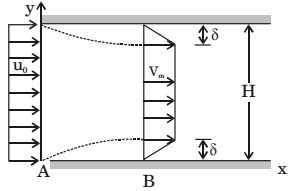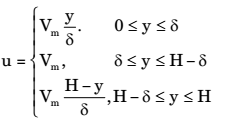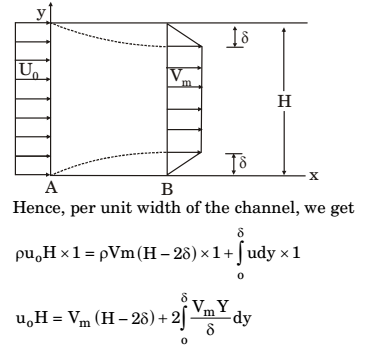## Fluid Mechanics and Hydraulic Machinery Miscellaneous

#### Fluid Mechanics and Hydraulic Machinery

1. Two pipes of uniform section but different diameters carry water at the same flow rate. Water properties are the same in the two pipes. The Reynolds number, based on the pipe diameter

1.  Reynolds number, Re = Vd υ

 Discharge, Q = AV = π d2V 4

 From above, = 4Q πd2

 ∴  Reynolds No. = 4Q × d πd2 2

 Reynolds No. = 4Q ....(1) πd

 From (1), Re ∝ 1 2

Reynolds number is large in the Narrow pipe.

##### Correct Option: B

 Reynolds number, Re = Vd υ

 Discharge, Q = AV = π d2V 4

 From above, = 4Q πd2

 ∴  Reynolds No. = 4Q × d πd2 2

 Reynolds No. = 4Q ....(1) πd

 From (1), Re ∝ 1 2

Reynolds number is large in the Narrow pipe.

Direction: Consider a linear programming problem with two variable and two constraints. The objective function is maximize x1 + x2. The corner points of the feasible region are (0, 0), (0,2) (2, 0) and (4/3, 4/3)

1. Oil is being pumped through a straight pipe, the pipe length, diameter and volumetric flow rate are all doubled in a new arrangement. The pipe friction factor, however, remains constant. The ratio of pipe frictional losses in the new arrangement to that in the original configuration would be

1. For original configuration
l1 = l
d1 = d
Q1 = Q
For new configuration
l2 = 21
d2 = 2d
Q2 = 2Q

 hf = flV² 2gb

where f = friction factor
l = Length of pipe
v = Average velocity
d = Diameter pipe
 Discharge: Q = AV = π a²V 4

 or   V = 4Q πa²

 hf = fl ×4Q² = 8flQ² 2gd πa² πga5

For new Pipe,
 hf2 = 8fl1Q2² = 8f × 2f × 4Q² = 2fQ² πgda5 π × 25 × a5 πga5

 h1 = 2flQ² × πga5 = 1 h2² πga5 2flQ² 4

##### Correct Option: A

For original configuration
l1 = l
d1 = d
Q1 = Q
For new configuration
l2 = 21
d2 = 2d
Q2 = 2Q

 hf = flV² 2gb

where f = friction factor
l = Length of pipe
v = Average velocity
d = Diameter pipe
 Discharge: Q = AV = π a²V 4

 or   V = 4Q πa²

 hf = fl ×4Q² = 8flQ² 2gd πa² πga5

For new Pipe,
 hf2 = 8fl1Q2² = 8f × 2f × 4Q² = 2fQ² πgda5 π × 25 × a5 πga5

 h1 = 2flQ² × πga5 = 1 h2² πga5 2flQ² 4

1.  The ratio PA − PB . (1/2)ρu0²

(where pA and pB are the pressures at section A and B, respectively, and ρ is the density of the fluid), is

1. Applying Bernoulli’s equation at sections A and B, we get

 PA + u0² + ZA = PA + Vm² + ZB ρg 2g ρg 2g

 PA + u0² = PB + Vm² ρg 2g ρg 2g
 or PA + PB = Vm² + u0² Pg ρg 2g 2g

Now, the ratio
 PA − PB/(1/2)ρ0² = ρVm² − ρu0² 2 2 1 ρu0² 2

 = Vm² − u0² =Vm² − 1 u0² u0

 Substituting the Value of Vm = 1 u0 1 − (δ/H)

we get
 PA − PB = 1 − 1 (1/2)ρ0² [1 − (δ/H)]²

##### Correct Option: B

Applying Bernoulli’s equation at sections A and B, we get

 PA + u0² + ZA = PA + Vm² + ZB ρg 2g ρg 2g

 PA + u0² = PB + Vm² ρg 2g ρg 2g
 or PA + PB = Vm² + u0² Pg ρg 2g 2g

Now, the ratio
 PA − PB/(1/2)ρ0² = ρVm² − ρu0² 2 2 1 ρu0² 2

 = Vm² − u0² =Vm² − 1 u0² u0

 Substituting the Value of Vm = 1 u0 1 − (δ/H)

we get
 PA − PB = 1 − 1 (1/2)ρ0² [1 − (δ/H)]²

Direction: Consider a steady incompressible flow through a channel as shown below:The velocity profile is uniform with a value of u0 at the inlet section A. The velocity profile at section B downstream is1. The ratio Vm/u0 is

1. Applying continuity equation at sections A and B, mass flow rate at inlet section A = mass rate at section B.∴  u = VmYδ

 u0H = Vm(H − 2δ) + 2 VmY²δ δ 2 0

 u0H = Vm(H − 2δ) + 2 Vm × δ² δ 2

u0H = Vm(H − 2δ) + Vmδ
u0H = VmH − 2Vmδ + Vmδ
u0H = VmH − Vmδ
u0H = Vm(H − δ)
 or Vm = H = 1 u0 H − δ 1 − (δ/H)

##### Correct Option: C

Applying continuity equation at sections A and B, mass flow rate at inlet section A = mass rate at section B.∴  u = VmYδ

 u0H = Vm(H − 2δ) + 2 VmY²δ δ 2 0

 u0H = Vm(H − 2δ) + 2 Vm × δ² δ 2

u0H = Vm(H − 2δ) + Vmδ
u0H = VmH − 2Vmδ + Vmδ
u0H = VmH − Vmδ
u0H = Vm(H − δ)
 or Vm = H = 1 u0 H − δ 1 − (δ/H)

1. Consider steady laminar incompressible axisymmetric fully developed viscous flow through a straight circular pipe of constant crosssectional area at a Reynolds number of 5. The ratio of inertia force to viscous force on a fluid particle is

1.  Reynold’s number = Inertia force = 5 Viscous force

##### Correct Option: A

 Reynold’s number = Inertia force = 5 Viscous force